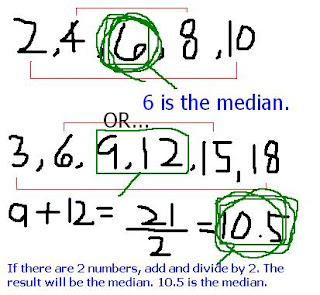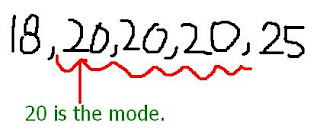### Hanbit's Measures of Central Tendency

Monday, September 29, 2008
Mean:
• Arrange data in ascending order.
• Sum of all data, divided by how many numbers there are in the dataMedian:

• Arrange data in ascending order.
• If there are 2 medians, add them together, then divide by 2 to get the average.
• The middle number from the dataMode:

• Arrange data in ascending order.
• The most common numbers in the data.Range

• Arrange data in ascending order.
• subtract the smallest piece of data from the greatest piece of data.Here is a video from youtube that explains mean, median, mode and range.